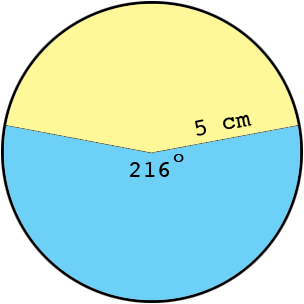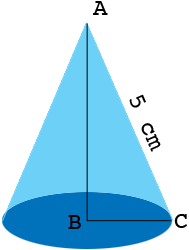SEARCH HOMEMath Central Quandaries & QueriesQuestion from Tinashe: A 216 sector of a circle of radius 5cm is bent to form a cone. Find the radius of the base of the cone and its vertical angle.Hi Tinashe,

The circumference of a circle is $\pi$ times the diameter so in your circle the circumference is $10 \times \pi.$Thus the $216^o$ sector of this circle, shaded blue, is subtended by an arc of length

$\frac{216}{360} \times 10 \times \pi \mbox{ centimeters}$

If you roll up the blue sector in the diagram above to form a cone then the arc of length

$\frac{216}{360} \times 10 \times \pi \mbox{ centimeters}$

forms the circumference of the base circle of the cone.Use the formula for the circumference of a circle to find the radius, |BC|, of the base circle. Use the triangle ABC to determine the vertical angle.

Penny* Registered trade mark of Imperial Oil Limited. Used under license.Math Central is supported by the University of Regina and the Imperial Oil Foundation.